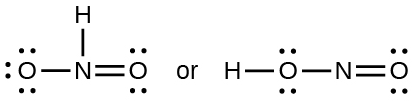# Problem: Which of the following structures would we expect for nitrous acid? Determine the formal charges:

###### FREE Expert Solution

We’re being asked to determine the best structure of nitrous acid by determining the formal charges of the atoms present.

To do so, we need to do the following steps:

Step 1: Determine the central atom in the molecule.

Step 2: Calculate the total number of valence electrons present.

Step 3: Draw the Lewis structure for the molecule.

Step 4: Calculate the formal charge for the indicated atom.

Recall that the formula for the formal charge is:

89% (330 ratings)###### Problem Details

Which of the following structures would we expect for nitrous acid? Determine the formal charges: